Download PresentationSolving Review Semester 2

# Solving Review Semester 2 - PowerPoint PPT Presentation

Solving Review Semester 2. Notice the variable is in the exponent. That means we need to use logs to solve. Because an “e” is involved we must use ln. Take the ln of both sides. Because it is now in ln form AND because of the power property of logs we can move the “x-1” down in front.I am the owner, or an agent authorized to act on behalf of the owner, of the copyrighted work described.
Download Presentation## Solving Review Semester 2

An Image/Link below is provided (as is) to download presentation

Download Policy: Content on the Website is provided to you AS IS for your information and personal use and may not be sold / licensed / shared on other websites without getting consent from its author.While downloading, if for some reason you are not able to download a presentation, the publisher may have deleted the file from their server.

- - - - - - - - - - - - - - - - - - - - - - - - - - E N D - - - - - - - - - - - - - - - - - - - - - - - - - -
Presentation Transcript### Solving ReviewSemester 2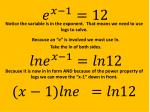Notice the variable is in the exponent. That means we need to use logs to solve.

Because an “e” is involved we must use ln.

Take the ln of both sides.

Because it is now in ln form AND because of the power property of logs we can move the “x-1” down in front.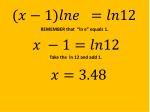REMEMBER that “ln e” equals 1.

Take the ln 12 and add 1.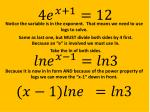Notice the variable is in the exponent. That means we need to use logs to solve.

Same as last one, but MUST divide both sides by 4 first.

Because an “e” is involved we must use ln.

Take the ln of both sides.

Because it is now in ln form AND because of the power property of logs we can move the “x-1” down in front.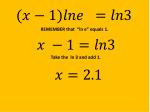REMEMBER that “ln e” equals 1.

Take the ln 3 and add 1.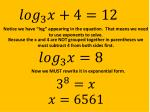Notice we have “log” appearing in the equation. That means we need to use exponents to solve.

Because the x and 4 are NOT grouped together in parentheses we must subtract 4 from both sides first.

Now we MUST rewrite it in exponential form.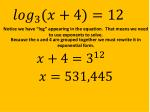Notice we have “log” appearing in the equation. That means we need to use exponents to solve.

Because the x and 4 are grouped together we must rewrite it in exponential form.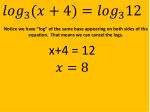Notice we have “log” of the same base appearing on both sides of the equation. That means we can cancel the logs.

x+4 = 12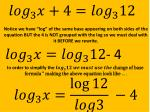Notice we have “log” of the same base appearing on both sides of the equation BUT the 4 is NOT grouped with the log so we must deal with it BEFORE we rewrite.

- 4

In order to simplify the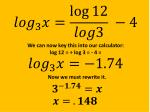We can now key this into our calculator:

log 12 = ÷ log 3 = - 4 =

Now we must rewrite it.) = 5

Notice we have “log” of the same base appearing on the SAME side of the equation.

WE CANNOT CANCEL THE LOGS!

We must instead rewrite the equation in terms of a single log using the log properties.

) = 5

Now we must rewrite into exponential formSince we get a negative under the square root there is no solution.Square both sides to get rid of the square root. Don’t forget to FOIL the “ x – 2”

After moving the x and -4 over we either factor or use quadratic formula.We raise both sides to the

=

T

(x +2)= 8

x = 6=

Eliminate the denominators by multiplying each of the three terms by the lowest common denominator which is x(x+2)

x(x+2) = x(x+2)

Cancel….

x(x+2) = x(x+2)

4x = 5(x+2)4x = 5(x+2)

Distribute, collect like terms, set equal to zero and use factoring or quadratic formula.

4x = 5

0 =

Since we get a negative under the square root there is no solution.=

Eliminate the denominators by multiplying each of the three terms by the lowest common denominator BUT to find the LCD we must factor FIRST, (x+2)(x-4). The LCD is then (x+2)(x-4)

(x -4)(x+2) = (x-4)(x+2)

Cancel….

(x -4)(x+2) = (x-4)(x+2)4(x – 4) = 5 +

Distribute, collect like terms, set equal to zero, factor or use quadratic formula.

4x – 16 = 5 +

4x – 16 =

0=Notice that this is a cubic. You hope that you can solve it by taking out an x from each term but you cannot because the last term has no x.

You hope that it has FOUR terms because then you might be able to solve by grouping the first two factors and the last two factors and solve by factoring. You cannot since there are only 3 terms.

You have to find the p’s and q’s technique. Remember that the coefficient of the cubed term is the q and the 12 is the p term.

Step 1: Factor 12. Remember that we have to include the negative and positive factors.

± 1, ±2, ±3, ±4, ±6, ±12

Step 2: Now using synthetic division we see which factor divides evenly into the polynomial.Trial # 1: x = 1

1

1

8

19

12

28

1

9

Remember that if this doesn’t result in a 0 then x = 1 IS NOT a factor.

28

1

9

40Trial # 2: x = -1

-1

1

8

19

12

-12

-1

-7

Because the result is a 0 then x = -1 IS a factor.

12

1

7

0

Now we rewrite into a quadratic and either solve using quadratic formula or factoring.

(x + 3)(x + 4)

That means the solutions are: -1,-3, -4